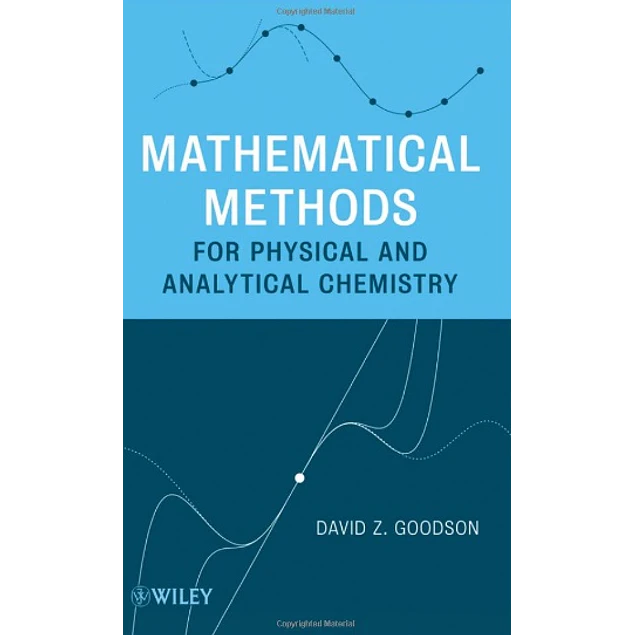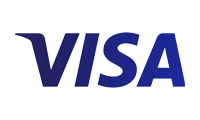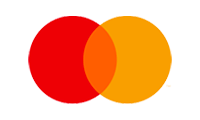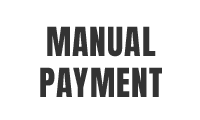## Mathematical Methods for Physical and Analytical Chemistry

10.00\$# Mathematical Methods for Physical and Analytical Chemistry

10.00\$

1st Edition

by David Z. Goodson (Author)

Mathematical Methods for Physical and Analytical Chemistry presents mathematical and statistical methods to students of chemistry at the intermediate, post-calculus level. The content includes a review of general calculus; a review of numerical techniques often omitted from calculus courses, such as cubic splines and Newton’s method; a detailed treatment of statistical methods for experimental data analysis; complex numbers; extrapolation; linear algebra; and differential equations. With numerous example problems and helpful anecdotes, this text gives chemistry students the mathematical knowledge they need to understand the analytical and physical chemistry professional literature.

Year:
2011
Pages:
389
Language:
English
Format:
PDF
Size:
21 MB
ISBN-10:
470473541
ISBN-13:
978-0470473542
##### Contact
•••••